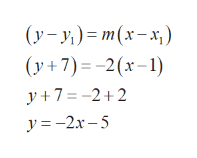# Find the slope and the equation of the tangent line to the graph of the function at the given point. f(x)= -2x +5 (-1,7)

Question
2 views

Find the slope and the equation of the tangent line to the graph of the function at the given point.

f(x)= -2x +5 (-1,7)

check_circle

Step 1

Given,

f(x)= -2x +5 and the point (1-,7)

Step 2

The slope of the tangent line is given by its first derivative of  f(x),

Step 3

Therefore, the equation of the tangent line passing through (x1,y1)=(1,-7) and has ...help_outlineImage Transcriptionclose(y– y,) = m(x-x;) y-. (y+7)=-2(x-1) y + 7 = -2+2 y =-2x – 5 fullscreen

### Want to see the full answer?

See Solution

#### Want to see this answer and more?

Solutions are written by subject experts who are available 24/7. Questions are typically answered within 1 hour.*

See Solution
*Response times may vary by subject and question.
Tagged in

### Other